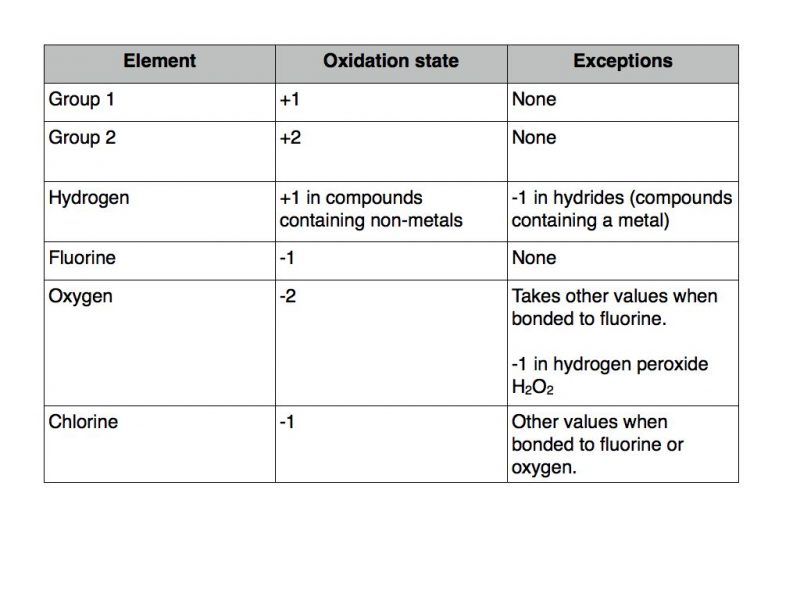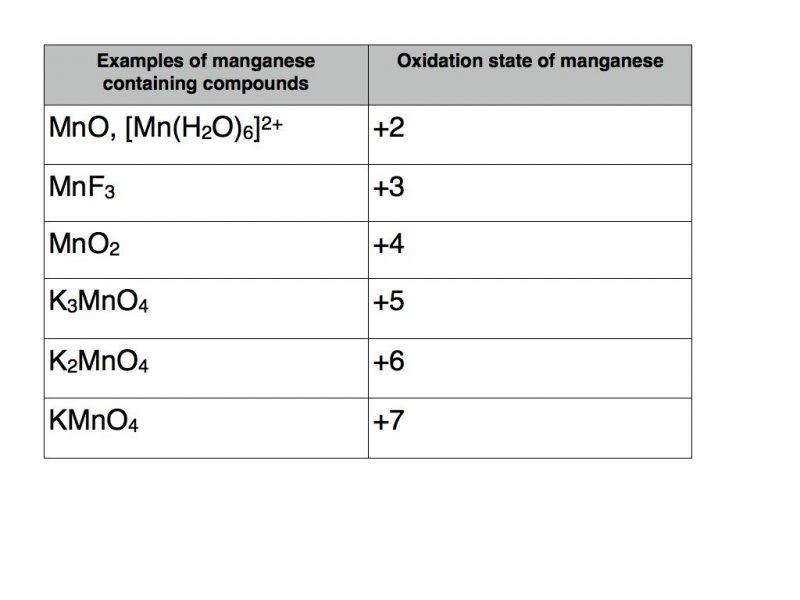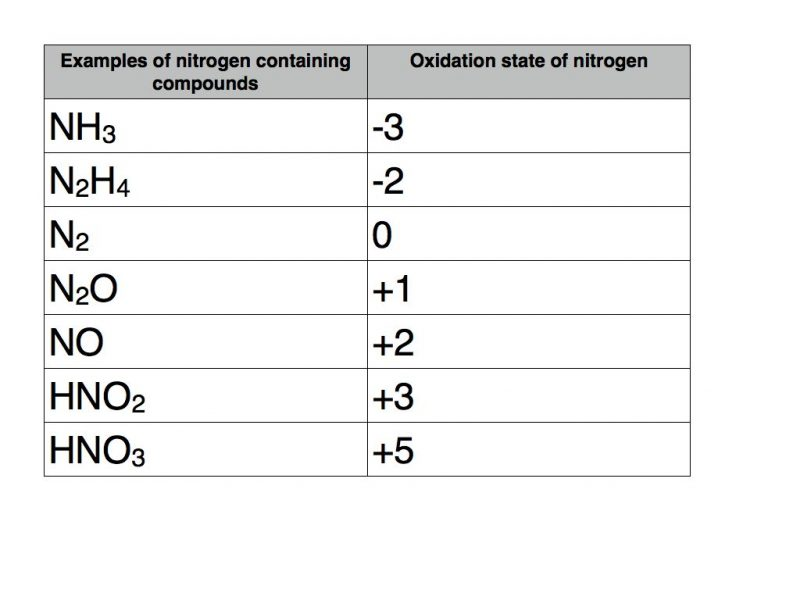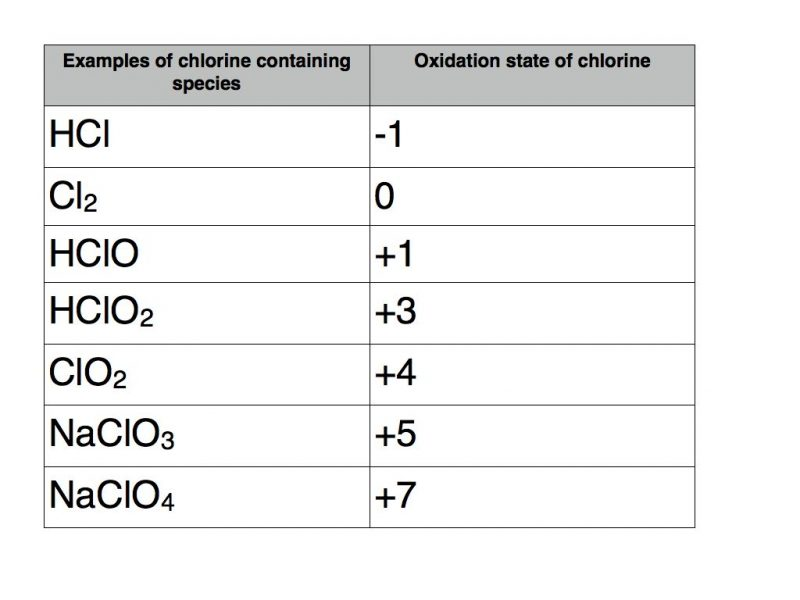In my last blog post I introduced the concept of oxidation state.  In this blog post you will find a wide variety of worked examples.

The following set of rules should be applied.## Ionic compounds

### Metals in group 1, group 2 and aluminium

The metal will form the positive ion corresponding to the group number as this is the number of electrons in the outer electron shell.  The non metal forms a negative ion with charge equal to the number of electrons required to fill its outer electron shell.

Take magnesium sulphide (MgS) as an example.  Magnesium is in group 2 so it will have an oxidation state of +2.  The compound has no overall charge so the oxidation state of sulphur must be -2.

However in magnesium sulphate (MgSO4) because oxygen must take an oxidation state of -2, sulphur must have a positive number.  In this case sulphur has the oxidation state +6 in order to balance the total oxidation number of -8 from four oxygen atoms.  Magnesium is, of course, still +2.  The full name of this compound is magnesium sulphate (VI).

### Compounds containing a transition metal

Transition metals are renowned for their ability to take a variety of oxidation states.  A classic example is the element manganese.

The table below contains a number of examples of manganese containing compounds with oxidation states varying from +2 to +7.The usual rules apply as far as the other elements are concerned.  Therefore potassium takes oxidation state +1, fluorine -1, oxygen -2 and hydrogen +1.

The example in square brackets is known as a transition metal complex.  It contains six water molecules with coordinate bonds to manganese.  As each water molecule has a total oxidation number of zero they do not contribute to the +2 total oxidation number of the complex which must therefore be solely attributable to manganese.

### Oxidation numbers of nitrogen

Nitrogen can take a variety of oxidation numbers depending upon whether it is bonded to elements that are more or less electronegative than itself.  The following table contains a number of examples.Nitrogen can take any oxidation state between -3 and +5.

A useful rule of thumb for p block elements that exhibit variable oxidation states is that the range of possible oxidation states is between the group number and the group number minus eight.

### Oxidation numbers of chlorine

Applying this rule of thumb to chlorine would indicate that it can take any oxidation state between -1 and +7.  But don’t forget that in a compound it will be -1 unless it is bonded to oxygen of fluorine.  Here are some examples.I hope you have found my oxidation state examples helpful.  If you need additional assistance, or have an idea for my next blog  post then please contact me via email, twitter or Facebook.